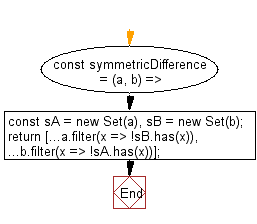# JavaScript: Get the symmetric difference between two given arrays

## JavaScript fundamental (ES6 Syntax): Exercise-134 with Solution

Write a JavaScript program to get the symmetric difference between two given arrays.

• Create a new Set() from each array to get the unique values of each one.
• Use Array.prototype.filter() on each of them to only keep values not contained in the other.

Sample Solution:

JavaScript Code:

``````//#Source https://bit.ly/2neWfJ2
const symmetricDifference = (a, b) => {
const sA = new Set(a),
sB = new Set(b);
return [...a.filter(x => !sB.has(x)), ...b.filter(x => !sA.has(x))];
};

console.log(symmetricDifference([1, 2, 3], [1, 2, 4]));
```
```

Sample Output:

```[3,4]
```

Pictorial Presentation:Flowchart:Live Demo:

See the Pen javascript-basic-exercise-134-1 by w3resource (@w3resource) on CodePen.

Improve this sample solution and post your code through Disqus

What is the difficulty level of this exercise?

Test your Programming skills with w3resource's quiz.

﻿

## JavaScript: Tips of the Day

Chunks an array into n smaller arrays

Example:

```const tips_chunkIntoN = (arr, n) => {
const size = Math.ceil(arr.length / n);
return Array.from({ length: n }, (v, i) =>
arr.slice(i * size, i * size + size)
);
}
console.log(tips_chunkIntoN([1, 2, 3, 4, 5, 6, 7,8], 4));
```

Output:

```[[1,2],[3,4],[5,6],[7,8]]
```